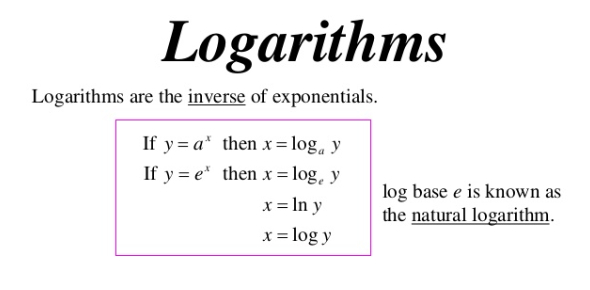# Logarithm Trivia Quiz! Practice Test Questions!

8 Questions | Total Attempts: 8899SettingsWhat do you know about logarithms? Do you know enough to pass this test? In mathematics, the logarithm is the inverse function of exponentiation. That means that the logarithm of a given number x is the exponent to which another fixed number, the base b, must be raised to produce that number x. Take the logarithm quiz and see how much you know about the logarithm.

• 1.
Logarithm properties must have the same base to be simplified.
• A.

True

• B.

False

• 2.
Rewrite 34 = 81 in logarithmic form.
• A.

Log34 = 81

• B.

Log813 = 4

• C.

Log381 = 4

• D.

Log481 = 3

• 3.
Change to Exponential Form: log636 = 2
• A.

26=36

• B.

62=36

• C.

362=6

• D.

366=2

• 4.
Rewrite logpt = m in exponential form.
• A.

Pt = m

• B.

Tm = p

• C.

Mt = p

• D.

Pm = t

Related TopicsBack to top